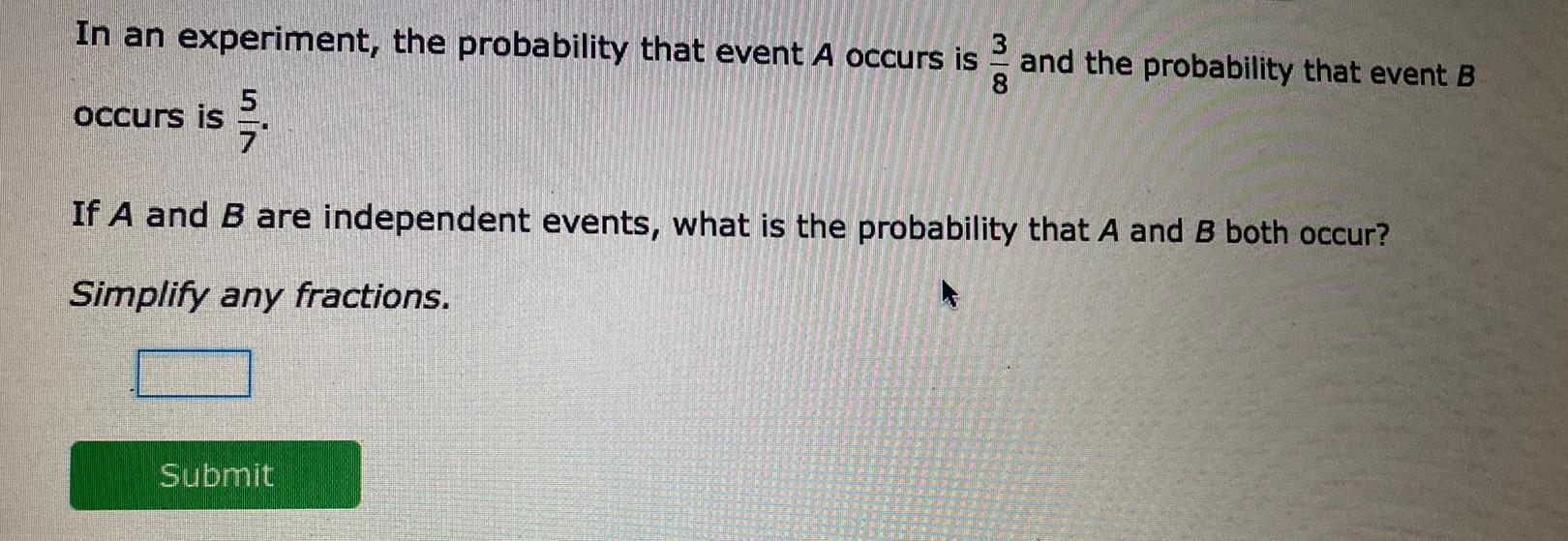Question:

# In an experiment the probability that event A occurs is 7

Last updated: 9/2/2023In an experiment the probability that event A occurs is 7 occurs is 38 Submit and the probability that event B If A and B are independent events what is the probability that A and B both occur Simplify any fractions